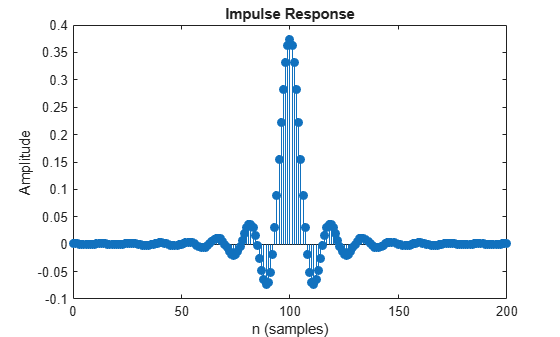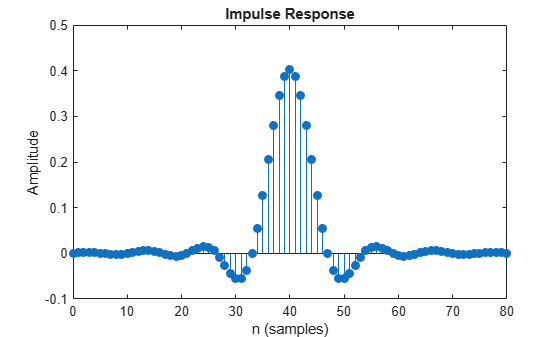coeffs

Coefficients for filters

Description

coefInfo = coeffs(rcfilter) obtain the coefficients for the specified filter System object™.

example

coefInfo = coeffs(rcfilter,'Arithmetic',arithType) analyzes the filter System object based on the arithmetic specified in arithType.

Examples

collapse all

Create a receive raised cosine filter and obtain its numerator coefficients.

srx = coeffs(rxfilter)
srx = struct with fields:
Numerator: [9.0942e-04 8.5589e-04 6.1364e-04 2.3196e-04 -2.0735e-04 ... ]

Calculate the expected number of numerator coefficients and confirm the value equals the length of srx.Numerator.

numcoefs = rxfilter.FilterSpanInSymbols * rxfilter.InputSamplesPerSymbol + 1
numcoefs = 201
isequal (numcoefs,length(srx.Numerator))
ans = logical
1

Display the first ten coefficients.

srx.Numerator(1:10)
ans = 1×10
10-3 ×

0.9094    0.8559    0.6136    0.2320   -0.2074   -0.6071   -0.8759   -0.9486   -0.8021   -0.4623

Display the impulse response of the receive raised cosine filter.

fvtool(rxfilter,'impulse')Create a transmit raised cosine filter and obtain its numerator coefficients.

txfilter = comm.RaisedCosineTransmitFilter('RolloffFactor',0.5);
stx = coeffs(txfilter);

Display the first ten filter coefficients.

stx.Numerator(1:10)
ans = 1×10

-0.0002    0.0011    0.0021    0.0024    0.0018    0.0004   -0.0014   -0.0029   -0.0036   -0.0031

Display the impulse response of the transmit raised cosine filter.

fvtool(txfilter,'impulse')Input Arguments

collapse all

Input filter, specified as one of these of filter System objects.

Arithmetic used in the filter analysis, specified as 'double', 'single', or 'Fixed'. When you do not specify the arithmetic type and the filter System object is unlocked, the analysis tool assumes a double-precision filter. When you do not specify the arithmetic type and the System object is locked, the function performs the analysis based on the data type of the locked input.

The 'Fixed' value applies to filter System objects with fixed-point properties only.

When you specify this input as 'Fixed' and the filter object has the data type of the coefficients set to 'Same word length as input', the arithmetic analysis performed depends on whether the System object is unlocked or locked.

• If the System object is unlocked, the analysis object function cannot determine the data type of the coefficients. The function assumes that the data type of the coefficients is signed, has a 16-bit word length, and is autoscaled. The function performs fixed-point analysis based on this assumption.

• If the System object is locked –– When the input data type is 'double' or 'single', the analysis object function cannot determine the data type of the coefficients. The function assumes that the data type of the coefficients is signed, has a 16-bit word length, and is autoscaled. The function performs fixed-point analysis based on this assumption.

To check if the System object is locked or unlocked, use the isLocked function.

When you specify this input as 'Fixed' and the filter object has the data type of the coefficients set to a custom numeric type, the object function performs fixed-point analysis based on the custom numeric data type.

Data Types: char | string

Output Arguments

collapse all

Filter coefficient information, returned as a structure containing the filter coefficients in the Numerator field. When the filter uses fixed-point arithmetic, the function returns additional information about the filter. This information includes the arithmetic setting and details about the filter internals.